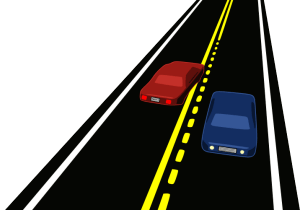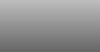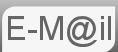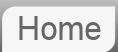# Answer to Puzzle #38: Probability of Seeing a Car in 10 Minutes & 30 Minutes

38. On a deserted road, the probability of observing a car during a thirty-minute period is 95%. What is the chance of observing a car in a ten-minute period?

Yet another puzzle sent to me not through the site but from a friend:Before reading the answer can I interest you in a clue?

To clarify the question we are saying the probability of seeing any other cars in 30 minutes is 95% or more clearly, and more usefully, the probability of not seeing any other cars is 5%.

As is always the case in the abscence of any other information we will make assumptions, in this case that the cars are randomly distributed. In questions like this it is generally taken as a given, but you may be asked to explicity state your assumptions.

In order that we do not see a car for 30 minutes it is necessary that we don't see another car for 10 minutes 3 times in a row. Just as the probability of tossing a coin and getting 3 heads in a row is given by ½^3

P(not30)=P(not10)^3

Where P(not30) and P(not10) are the probabilities of not seeing a car in 30 and 10 minutes respectively. We know P(not30) is 5% or 0.05 and the equation is now solvable by taking the cube root of 0.05

P(not10) = cube root (0.05) = 3√0.05 = 0.3684 roughly

Remember the question asked what is the probability of seeing a car in 10 minutes, we have worked out the probability of not seeing a car so we need 1 - 0.3684

The chance of observing a car in a ten-minute period is .6316 roughly.

© Nigel Coldwell 2004 -  – The questions on this site may be reproduced without further permission, I do not claim copyright over them. The answers are mine and may not be reproduced without my expressed prior consent. Please inquire using the link at the top of the page. Secure version of this page.

PayPal
I always think it's arrogant to add a donate button, but it has been requested. If I help you get a job though, you could buy me a pint! - nigel